# Chaos Theory and the Logistic Map¶

In this tutorial, we will see how to implement Geoff Boeing's excellent blog post on Chaos Theory and the Logistic Map using our newly release library, HoloViews. For an example of how this material may be approached using pandas and matplotlib directly please see Geoff's original notebook.

We will see how using HoloViews allows the same content to be expressed in a far more succinct way that also makes the material presented easier to understand for the reader. In particular, we will show how composing Layout and Overlay objects makes it easier to compare data side-by-side, without needing to scroll vertically between plots.

We now start by importing numpy and the classes we need from HoloViews before loading the IPython extension:

In :
import numpy as np
import holoviews as hv
from holoviews import Dimension
hv.notebook_extension()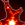HoloViewsJS successfully loaded in this cell.

## The Logistic Model¶

Here we define a very simple logistic_map function that is defined by the difference equation $x_{t+1} = rx_t(1-x_t)$. This is the logistic map, a very simple model of population dynamics with chaotic behavior:

In :
def logistic_map(gens=20, init=0.5, growth=0.5):
population = [init]
for gen in range(gens-1):
current = population[gen]
population.append(current * growth * (1 - current))
return population


We will shortly look a few curve plots of this function, but before we do we will declare that all Curve objects will be indexed by the 'Generation' as the key dimension (i.e x-axis) with a corresponding 'Population' calue dimension for the y-axis:

In :
hv.Curve.kdims =   [Dimension('Generation')]
hv.Curve.kdims = [Dimension('Population')]


Now with the NdOverlay class, we can quickly visualize the population evolution for different growth rates:

In :
%%opts Curve (color=Palette('jet')) NdOverlay [figure_size=200 aspect=1.5 legend_position='right']
hv.NdOverlay({growth: hv.Curve(enumerate(logistic_map(growth=growth)))
for growth in [round(el, 3) for el in np.linspace(0.5, 3.5, 7)]},
label = 'Logistic model results, by growth rate',
kdims=['Growth rate'])

Out: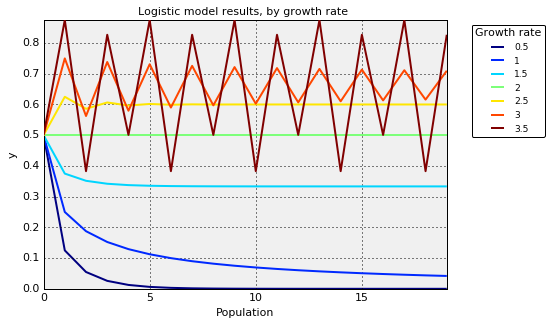As described in the original tutorial we see examples of population collapse, one perfectly static population trace and irregular oscillations in the population value.

## Bifurcation diagrams¶

Now we will plot some bifurcation diagrams using the Points class. We will lower the default Points size to $1$ and set the dimension labels as we did for Curve:

In :
%opts Points (s=1) {+axiswise}
hv.Points.kdims = [Dimension('Growth rate'), Dimension('Population')]


Now we look at the set of population values over growth rate:

In :
growth_rates = np.linspace(0, 4, 1000)

p1 = hv.Points([(rate, pop) for rate in growth_rates for
(gen, pop) in enumerate(logistic_map(gens=100, growth=rate))
if gen!=0])  # Discard the initial generation (where population is 0.5)

p2 = hv.Points([(rate, pop) for rate in growth_rates for
(gen, pop) in enumerate(logistic_map(gens=200, growth=rate))
if gen>=100])  # Discard the first 100 generations to view attractors
+ p2.relabel('Discarding the first 100 generations') + (p1 * p2).relabel('Overlay of B on A'))

Out: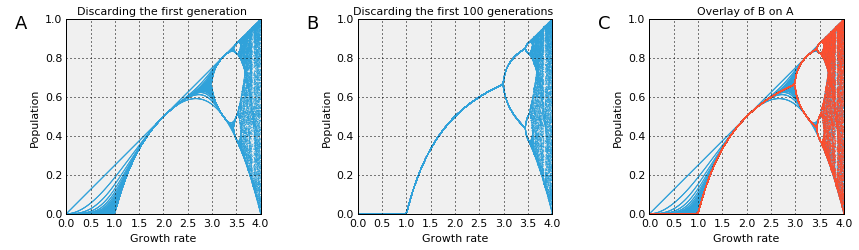In plot A, only discarding the initial population value is discarded. In B, the initial hundred population values are discarded. In C we overlay B on top of A to confirm that B is a subset of A.

Note how HoloViews makes it easy to present this information in a compact form that allows immediate comparison across subfigures with convenient sub-figure labels to refer to.

## Looking at chaos in more detail¶

Now we will zoom in on the first bifurcation point and examine a chaotic region in more detail:

In :
growth_rates = np.linspace(2.8, 4, 1000)
p3 = hv.Points([(rate, pop) for rate in growth_rates for
(gen, pop) in enumerate(logistic_map(gens=300, growth=rate))
if gen>=200])

growth_rates = np.linspace(3.7, 3.9, 1000)
p4 = hv.Points([(rate, pop) for rate in growth_rates for
(gen, pop) in enumerate(logistic_map(gens=200, growth=rate))
if gen>=100])
(p3 * p4) + p4

Out: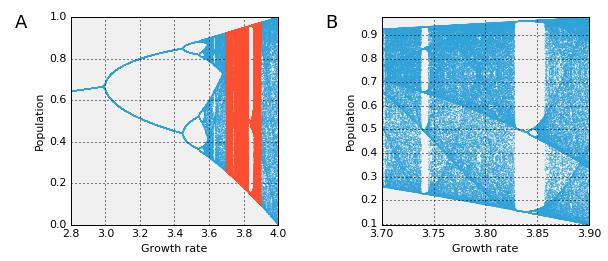Again, we use an Overlay to view the region of A expanded in B. Note that the declaration of +axiswise at the start of this section has decoupled the axes in A and B. Try setting -axiswise in the %opts declaration above to see A and B in directly comparable coordinate spaces.

## Self-similarity¶

The next part of the tutorial looks at self-similarity, by zooming into a small portion of the first bifurcation diagram shown above:

In :
growth_rates = np.linspace(3.84, 3.856, 1000)
p5 = hv.Points([(rate, pop) for rate in growth_rates for
(gen, pop) in enumerate(logistic_map(gens=500, growth=rate))
if gen>=300])[:, 0.445:0.552]
(p1 * p5) + p5

Out: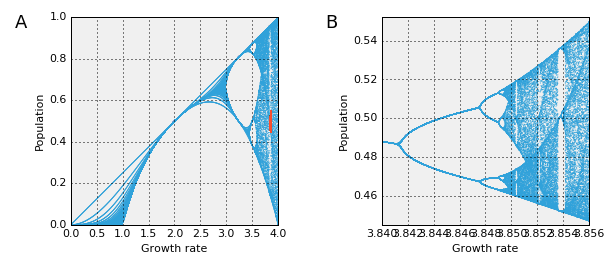Here we see qualitatively similar patterns on two very different scales, demonstrating a nice example of self-similarity.

## Sensitivity to initial conditions¶

Chaotic systems are well known for their sensitive-dependence on initial conditions. Here we look at sensitivity to both the population growth rate and the initial population value:

In :
%%opts Curve {+axiswise} NdOverlay [aspect=1.5] Layout [figure_size=150]
plot1 = hv.NdOverlay({str(growth): hv.Curve(enumerate(logistic_map(gens=30, growth=growth)))
for growth in [3.9, 3.90001]},
kdims=['Growth rate'],
label = 'Sensitivity to the growth rate')

plot2 = hv.NdOverlay({str(init): hv.Curve(enumerate(logistic_map(gens=50, growth=3.9, init=init)))
for init in [0.5, 0.50001]},
kdims=['Initial population'],
label = 'Sensitivity to the initial conditions')
(plot1 + plot2)

Out: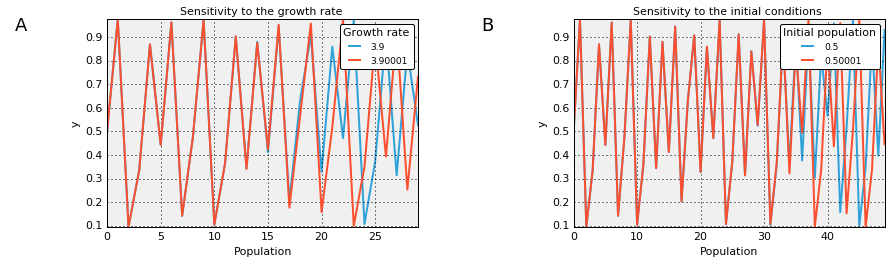In A we see how a tiny difference in the growth rate eventually results in wildly diverging behaviours. In B we see how tiny differences in the initial population value also eventually results in divergence.

In this example, we used a Layout container to place A next to B where each subfigure is an NdOverlay of Curve objects.

# Poincaré Plots¶

Now we will examine Poincaré plots for different growth rates. First, we will redefine the default dimensions associated with Points objects and set suitable, normalized soft-ranges:

In :
%opts NdLayout [title_format='Poincaré Plots']
hv.Points.kdims = [hv.Dimension('Population (t)',   soft_range=(0,1)),
hv.Dimension('Population (t+1)', soft_range=(0,1))]


Now we use an NdLayout to view the Poincaré plots for four different growth rates:

In :
%%opts Points (s=5)
layout = hv.NdLayout({rate: hv.Points(zip(logistic_map(gens=500, growth=rate)[1:],
logistic_map(gens=500, growth=rate)[2:]))
for rate in [2.9, 3.2, 3.5, 3.9]}, key_dimensions=['Growth rate']).cols(2)
layout

Out: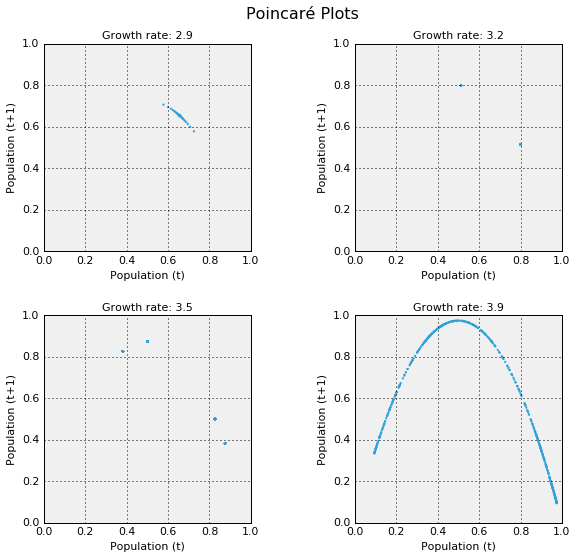As the chaotic regime is the most interesting, let's look at the Poincaré plots for 50 growth values equally spaced between $3.6$ and $4.0$. To distinguish all these curves, we will use a Palette using the 'hot' color map:

In :
%%opts NdOverlay [show_legend=False figure_size=200] Points (s=1 color=Palette('hot'))
hv.NdOverlay({rate: hv.Points(zip(logistic_map(gens=300, growth=rate)[1:],
logistic_map(gens=300, growth=rate)[2:]), extents=(0.0001,0.0001,1,1))
for rate in np.linspace(3.6, 4.0, 50)}, key_dimensions=['Growth rate'])

Out: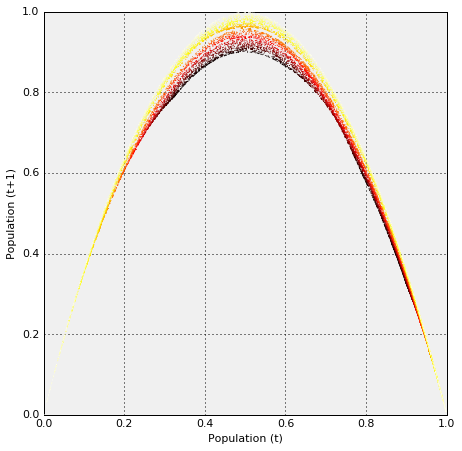What is fascinating about this family of parabolas is that they never overlap; otherwise two different growth rates starting with the same intial population would end up with identical evolution. The logistic_map function is determinstic after all. This type of non-overlapping, non-repeating yet structured evolution is a general feature of fractal geometries. In the next section, we will constrast chaotic behaviour to random behaviour.

## Chaos versus randomness¶

At first glance, the evolution of a chaotic system can be difficult to tell apart from a set of samples drawn from a random distribution:

In :
%%opts NdOverlay [figure_size=200 aspect=1.5]
chaotic = hv.Curve([(gen, pop) for gen, pop in enumerate(logistic_map(gens=100, growth=3.999))],
kdims=['Generation'])[40:90]
random = hv.Curve([(gen, np.random.random()) for gen in range(0, 100)],
kdims=['Generation'])[40:90]
hv.NdOverlay({'chaotic':chaotic, 'random':random},
label='Time series, deterministic versus random data')

Out: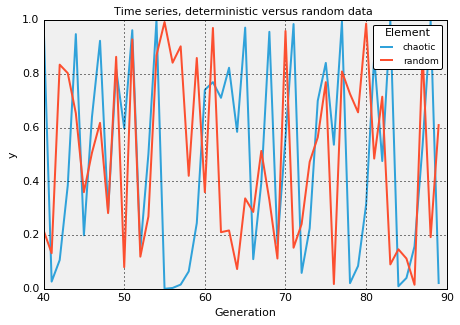The Poincaré plots from the previous section do provide a clear way of distinguishing chaotic evolution from randomness:

In :
randpoints = [np.random.random() for _ in range(0, 1000)]
poincare_random = hv.Points(zip(randpoints[1:], randpoints[2:]))
layout[3.9] + poincare_random

Out: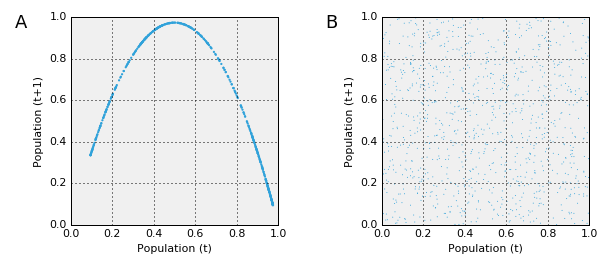In this example, we index into one element of the NdOverlay of Poincaré plots defined earlier (A) in order to contrast it to the random case shown in B. Using these plots, the two sources of data can be clearly distinguished.

## The 3D attractor¶

Finally, we generalize the 2D plots shown above into a three-dimensional space using the Scatter3D element, plotting the values at time $t$ against $t+1$ and $t+2$:

In :
%opts Scatter3D [title_format='3D Poincaré Plot'] (s=1 color='r') Layout [figure_size=200]
population = logistic_map(2000, 0.5, 3.99)
attractor3D = hv.Scatter3D(zip(population[1:], population[2:], population[3:]),
kdims=['Population (t)', 'Population (t+1)', 'Population (t+2)'])

random = [np.random.random() for _ in range(0, 2000)]
rand3D = hv.Scatter3D(zip(random[1:], random[2:], random[3:]),
kdims=['Value (t)', 'Value (t+1)', 'Value (t+2)'])
attractor3D + rand3D

Out: